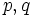# Character degree graph of a finite group

## Definition

Suppose$G$ is a finite group. The character degree graph of$G$, sometimes denoted$\Gamma(G)$, is defined as the following undirected graph:

1. Its vertex set is precisely the set of primes$p$ such that$p$ divides the degree of at least one irreducible representation of$G$ over$\mathbb{C}$ (we could replace$\mathbb{C}$ by any splitting field). Note that the precise vertex set can be determined using the Ito-Michler theorem from knowledge of the Sylow subgroup structure, without knowledge of the degrees of irreducible representations.
2. Its edge set is defined as follows: two distinct primes$p,q$ are adjacent if and only if the product$pq$ divides the degree of at least one irreducible representation of$G$ over$\mathbb{C}$ (we could replace$\mathbb{C}$ by any splitting field).

Note that the character degree graph is completely determined number-theoretically by the degrees of irreducible representations (also called the character degrees). In fact, it depends only on the set of numbers occurring as character degrees, without even caring for the number of irreducible representations of a given degree.

## Particular cases

Case on$G$ What can we conclude about the character degree graph? Explanation
finite abelian group empty graph (no vertices, no edges) abelian implies every irreducible representation is one-dimensional
non-abelian group of prime power order for some prime number$p$ one-point graph with point corresponding to$p$ degree of irreducible representation divides order of group, and the fact that there is at least one irreducible representation of degree greater than one
finite nilpotent group the graph is a complete graph with its vertex set equal to the set of primes$p$ for which the$p$-Sylow subgroup of$G$ is non-abelian By equivalence of definitions of finite nilpotent group, the group is a direct product of its Sylow subgroups. By degrees of irreducible representations of direct product are pairwise products of degrees of irreducible representations of direct factors, we obtain that the degrees are obtained by taking all possible products of degrees for the Sylow subgroups. This gives the indicated graph.